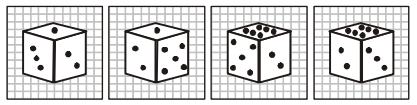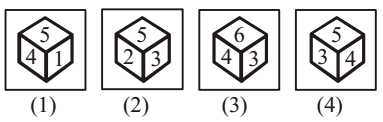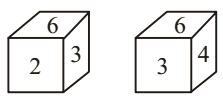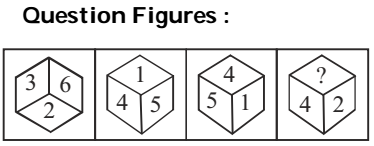## Cubes and Dices

#### Cubes and Dices

1. 4 different views of a cube/ dice are given as viewed from different angles. Find out the number of dots on the face opposite to the face with one dot.1. On the basis of question figure pattern ,
One dot, three dots, five dots and six dots is/are on the faces adjacent to two dots.
Therefore, four dots are on face opposite to two dots.
One dot, two dots and six dots is/are on the faces adjacent to three dots.

##### Correct Option: A

On the basis of question figure pattern ,
One dot, three dots, five dots and six dots is/are on the faces adjacent to two dots.
Therefore, four dots are on face opposite to two dots.
One dot, two dots and six dots is/are on the faces adjacent to three dots.
Therefore, five dots are on the face opposite to three dots.
Thus, six dots lie opposite one dot.

1. Study the 2 different positions of a cube given below with dots from 1 to 6 marked on its faces. Find out how many dots are contained on the face opposite to that containing 4 dots.1. On the basis of following pattern in given dices , we can say that
2, 4, 1 and 3 are adjacent to 5.
So 6 will be opposite to 5.
Now keeping position of 5 stable in both figures respectively.

##### Correct Option: C

On the basis of following pattern in given dices , we can say that
2, 4, 1 and 3 are adjacent to 5.
So 6 will be opposite to 5.
Now keeping position of 5 stable in both figures respectively.
4, 2 and 3 are in same order, hence 3 will be opposite to 4.

1. Four views of one cube are given below. Each of the six faces of cube is numbered. In figure 3 which number will be opposite to the face with number 3?1. As per the given pattern in question figure , we can find the similar next position of given dice .
The numbers 2, 4, 5 and 6 are on the adjacent faces of the number 3.

##### Correct Option: A

As per the given pattern in question figure , we can find the similar next position of given dice .
The numbers 2, 4, 5 and 6 are on the adjacent faces of the number 3.
Therefore, 1 lies opposite 3.

1. Two positions of a cube are given. Based on them find out which number is found opposite number 4 in a given cube?1. We follow the given pattern :-
Here 6 and 3 are common faces position .

##### Correct Option: B

We follow the given pattern :-
From the two views of the dice, it is clear that 2 lies opposite 4.

1. The following diagram depicts various views of a cube. Each faces has some number, where as in cube 4, one face is blank. From the answer choices select the number that should come in the blank space.1. On the basis of following pattern in given dices ,
From the four views of the cube it is clear that 4 lies opposite 6.
3 lies opposite 1.

##### Correct Option: D

On the basis of following pattern in given dices ,
From the four views of the cube it is clear that 4 lies opposite 6.
3 lies opposite 1.
2 lies opposite 5.
Therefore, the number 3 will be in the place of question mark.